# Collaborative Distribution in the Soft Time Window of Agricultural-Means Supply Chain Based on Simulated Annealing-Genetic Algorithm

Collaborative Distribution in the Soft Time Window of Agricultural-Means Supply Chain Based on Simulated Annealing-Genetic Algorithm
School of management, Heibei GEO University, Shijiazhuang 050031, China

Corresponding Author Email:
zhy2018@hgu.edu.cn
Page:
835-844
|
DOI:
https://doi.org/10.18280/jesa.530609
1 July 2020
|
Accepted:
5 October 2020
|
Published:
23 December 2020
| Citation

OPEN ACCESS

Abstract:

This paper mainly explores the collaborative distribution to multiple customers at the terminal of agricultural-means supply chain (AMSC). Firstly, a cost optimization model for collaborative distribution constrained by time window was constructed based on fuzzy appointment time function. Next, the proposed model was solved by simulated annealing-genetic algorithm (SA-GA). Through a case study, the cost optimization model constrained by customer satisfaction was compared with that not constrained by customer satisfaction. The results show that the cost optimization model constrained by customer satisfaction made the customers more satisfied without greatly elevating the distribution cost. The research results shed new light on the collaborative distribution of time-sensitive agricultural-means (AM) products, and the management of the AMSC.

Keywords:

agricultural-means supply chain (AMSC), collaborative distribution, soft time window, simulated annealing-genetic algorithm (SA-GA)

1. Introduction

The current studies on collaborative distribution mainly tackle three issues: the selection of distribution method, the selection, assembling, and loading of vehicles, and vehicle routing problem (VRP). Among them, the VRP has attracted much attention from the academia, owing to its complexity and wide application. Many mathematical models and solving algorithms have been established for this problem, providing an important guidance for distributors to improve the distribution efficiency .

In terms of model construction, Goodarzi and Zegordi  established a nonlinear mixed-integer programming (MIP) model to optimize the distribution network, consisting of parts suppliers, cross terminals, and assembly factories. Guedria et al.  optimized the urban vehicle routing and vehicle loading planning. To minimize the total cost of the collaborative multiple centers VRP (CMCVRP), Wang et al.  designed an integer programming model that considers the effective transport cost in distribution centers (DC) and that of vehicles from each DC, and solved the model with a self-designed multi-phase hybrid algorithm, which combines the merits of clustering, dynamic planning, and heuristic algorithms. Considering vehicle synchronization and grey zone customers, Alexandra et al.  constructed a multi-objective optimization model for two-level VRP in the context of urban freight deliveries.

Through practical applications and academic research, various forms of the VRP have emerged, such as the VRP with loading constraints [6, 7], the multi-VRP , the VRP with best service time [9, 10], the VRP with random or dynamic demand , and the green VRP [10, 12, 13]. In recent decades, there is a growing awareness of punctuality and customer satisfaction. As a result, more and more scholars begin to discuss the VRP with time constraints and the objective of customer satisfaction [13-15]. With the increase of model complexity, the solving algorithms of the VRP become increasingly diverse. The algorithms roughly fall into three categories: precise algorithms, traditional heuristic algorithms, and modern heuristic algorithms [16-18]. For instance, Hanafi et al.  presented a sweep algorithm to optimize the distribution routes in the capacitated VRP (CVRP).

To overcome the limitations of precise algorithms and traditional heuristic algorithms, modern heuristic algorithms have gained immense popularity, namely, genetic algorithm (GA), simulated annealing (SA) algorithm, tabu search (TS), and bionic algorithms [20-23]. To satisfy the time window, capacity limit, and order-vehicle compatibility, Sicilia et al.  put forward a meta-heuristic process algorithm that minimizes the distance and cost and optimizes the service quality. To minimize total cost and maximize freshness, Wang et al.  presented a multi-objective VRP with time window for perishable food (MO-VRPTW-P), and solved the problem with a two-stage heuristic algorithm: the GA based on Pareto variable neighborhood search considering time and space (STVNS-GA). Aibinu et al.  and Singh et al.  came up with a cluster-based GA for VRP. Li et al.  built up a mixed integer linear programming (MILP) model with simultaneous pickups and deliveries and time windows, and designed an intelligent water drop algorithm to solve the model.

To sum up, the long-term in-depth research into the VRP has created fruitful results on multi-objective models and solving algorithms, providing valuable reference for the terminal distribution of agricultural-means supply chain (AMSC). Agricultural-means (AM) products like fertilizers and pesticides are functional products with strong seasonality. The market demand for such products is greatly affected by the season and climate conditions, e.g., precipitation and temperature. The time-sensitivity demand fluctuates irregularly with the elapse of time. On the supply side, AM circulation enterprises pursue small profits but quick returns, due to the heavy investment, high logistics cost, and narrow profit margin of AM product circulation.

The supply and demand features of China’s AM pose a great challenge to the traditional stockpiling profit model of AM distributors and retailers. With the weakening effect of inventory, more and more enterprises have turned to improving transport and distribution abilities to cope with fluctuations in market demand. Nowadays, the AM distribution service becomes the key to the competition among AM circulation enterprises, for the social transport and distribution abilities have been enhanced by the improved transport infrastructure in rural areas and the leapfrog development of logistics across China.

So far, China has rolled out standards for AM products, and the AM producers have developed mature technologies, making AM products increasingly homogeneous. For most AM circulation enterprises, the only simulant of the farmers’ willingness to buy is services, especially logistics service. After all, their products are of similar quality and functions. Therefore, the service-oriented collaborative distribution with the objective of improving customer satisfaction has become an important aspect of AMSC management.

Considering the features of AM demand, this paper probes deep into the multi-retailer, multi-customer VRP of AMSC terminal distribution. Specifically, a cost optimization model was established for collaborative distribution constrained by time window, based on the fuzzy appointment time function. The model aims to minimize the distribution cost, while ensuring customer satisfaction. It is in line with the practice of AMSC management in China. The research results provide a reference for China’s AM circulation enterprises to enhance distribution ability, lower distribution cost, and improve customer satisfaction.

2. Cost Optimization Model for Collaborative Distribution

The distribution of AM products needs to satisfy the material and time demands of customers. In modern times, it is particularly important to distribute AM products on time. To solve the VRP for AM distribution, consideration should be given to the distribution cost, and the time window set by customers. Based on the actual situation of AM collaborative distribution, this paper decides to explore the VRP constrained by time window.

2.1 Time window and penalty function

During the design of distribution plan, the distribution enterprise can choose vehicles and routes with the least distribution cost, if customers have no requirements on distribution time, or the products are not time-sensitive. But time is a critical consideration in actual distribution. For example, the value of some products changes greatly with time. Many customers would set a specific distribution time for such products. Thus, the VRP is often turned into the VRP with time window (VRPTW).

Let Ti be the distribution time for the completion of task i. Then, the start time of task i must fall into the time window (ETi, LTi), where ETi and LTi are the earliest allowable start time and latest allowable start time of task i, respectively. If a vehicle is available before ETi, the vehicle must wait until ETi to execute task i; if no vehicle is available before LTi, task i must be postponed. That is, the time ti that a vehicle starts to execute task i must satisfy: ETitiLT.

There are three kinds of time windows in the VRPTW: hard time window (HTW), soft time window (STW), and mixed time window (MTW). The HTW means the products in each task must be delivered to customers within a time range. Neither early nor late delivery is acceptable. If a task is completed outside the range, a huge penalty will be imposed on the distribution enterprise. In this case, the solution is not feasible . The STW means, if a vehicle fails to complete task i, the distribution enterprise will face a penalty, which is positively correlated with the length of time violation. For example, if a vehicle completes task i after LTi, the penalty will be incurred by the service delay. Finally, the MTW means the distribution system is constrained by HTW and STW simultaneously.

Practices show that a large amount of distribution cost can be saved, if some customers accept service delay. For universality, this sub-section mainly studies the VRP with STW (VRPSTW). The penalty incurred by time violation was described by a cost function. In reality, the penalty curve may take different forms, such as exponential growth, and curvy growth. To simplify the problem, it is assumed that the penalty increases linearly. Hence, the cost function can be expressed as:

$C_{i}\left(t_{i}\right)=\left\{\begin{array}{ll}c_{1}\left(E T_{i}-t_{i}\right) & t_{i}<E T_{i} \\ 0 & E T_{i} \leq t_{i} \leq L T_{i} \\ c_{2}\left(t_{i}-L T_{i}\right) &t_{i}>L T_{i}\end{array}\right.$

where, Ci(ti) is the penalty imposed by customer i; ti is the arrival time of a vehicle at customer i; c1 the opportunity cost incurred by early arrival; c2 is the penalty incurred by late arrival. Figure 1 shows the penalty Ci(ti) curve.

## 1.png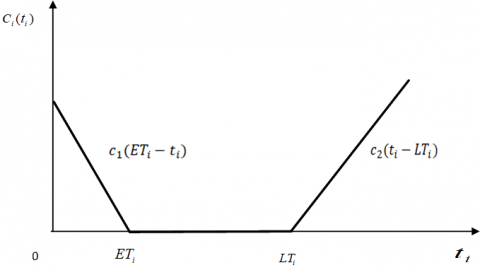Figure 1. The penalty Ci(ti) curve

Then, the total penalty Ci(ti) imposed by n customers can be calculated as: $C(t_{i})=c_{1} \sum_{i=1}^{n} \operatorname{Max}\left[\left(E T_{i}-t_{i}\right), 0\right]+c_{2} \sum_{i=1}^{n} \operatorname{Max}\left[\left(t_{i}-L T_{i}\right), 0\right]$.

2.2 Customer satisfaction based on fuzzy time

The distribution beyond the time window set by customers will bring a certain loss, including opportunity cost and penalty. To reduce the distribution cost, the distribution enterprise must arrange service time and distribution decisions in strict accordance with the time window.

The purpose of meeting the time window is to enhance customer satisfaction. To understand the changing customer demands, it is important to correctly evaluate the satisfaction level of customers. In general, customer satisfaction can be assessed by the following indices: the satisfaction rate of orders, the satisfaction of distribution price, and the satisfaction of service time.

Considering the features of customer demands for AM products, this sub-section mainly explores the collaborative distribution in the light of the satisfaction of service time. The fierce competition among AM suppliers provides farmers a dazzling array of choices. These customers pay attention to both distribution price and delivery timeliness.

Crop planting is sensitive to season and climate. For many farmers, the AM products need to be supplied within the short time window of planting. To grasp sales opportunities, the AM suppliers must strive to improve the timeliness and reliability of distribution service.

To reflect the supply-demand relationship in AM market, this sub-section discusses the collaborative distribution of AM products under the constraint of time window. As shown in Figure 2, customers are theoretically fully satisfied with services between ETi and LTi, and completely dissatisfied if the delivery is outside the time window.

## 2.png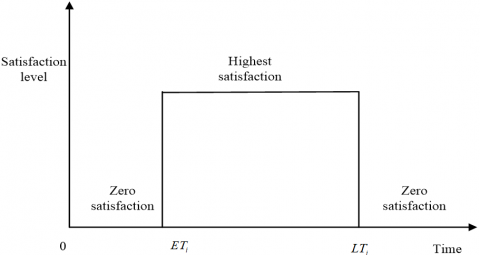Figure 2. The theoretical relationship between customer satisfaction and delivery time

In reality, however, the customer satisfaction varies with the specific time of delivery in the time window. Some customers might only be satisfied with the service provided in a short period within the time window. If the service is provided in other periods, the customer satisfaction could decrease as the delivery time moves away from the short period of full satisfaction.

Let [ETi, LTi] be the time window set by a customer, and [ETi*, LTi*] be the period of full satisfaction. As shown in Figure 3, the customer satisfaction increases in (ETi, ETi*), and decreases in (LTi*, LTi).

## 3.png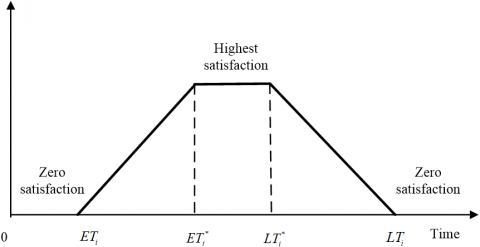Figure 3. The actual relationship between customer satisfaction and delivery time

Therefore, the satisfaction of customer i with the service time tican be described by a fuzzy appointment time function fi(ti):

$f_{i}\left(t_{i}\right)=\left\{\begin{array}{l}0 \\ \alpha \times\left(t_{i}-E T_{i}\right) /\left(E T_{i}^{*}-E T_{i}\right)+(1-\alpha) \\ 1 \\ \alpha \times\left(L T_{i}-t_{i}\right) /\left(L T_{i}-L T_{i}^{*}\right)+(1-\alpha) \\ 0\end{array}\right.$

$t_{i}<E T_{i}$

$E T_{i}<t_{i}<E T_{i}^{*}$

$E T_{i}^{*} \leq t_{i} \leq L T_{i}^{*}, t_{i}=E T_{i}=E T_{i}^{*}, t_{i}=L T_{i}=L T_{i}^{*}$

$L T_{i}^{*}<t_{i}<L T_{i}$

$t_{i}>L T_{i}$

where, ti is the arrival time of a vehicle at customer i; [ETi, LTi] is the time window required by customer i; [ETi*, LTi*] is the period of full satisfaction of customer i; α is the sensitivity of customer i to the time window and the period of full satisfaction.

2.3 Model construction

To reduce distribution cost and enhance customer satisfaction, the AM products should be delivered within the time window and in the quantity required by customers. Considering the dispersion and limited demand of each customer in rural market, both vehicle and route should be optimized to complete the distribution tasks in the corresponding time windows. Thus, this paper sets up a VRPSTW model, with customer satisfaction fi(ti) as the constraint, and the distribution cost DC, which is composed of effective transport cost FC and penalty C(ti), as the objective function:

$\operatorname{Min} \sum_{i=0}^{n} \sum_{j=0}^{n} \sum_{k=1}^{m}\left(C_{i j} d_{i j} \cdot x_{i j k}\right)+C\left(t_{i}\right)$      (1)

s.t. $\frac{\sum_{i=1}^{n} f_{i}\left(t_{i}\right)}{n} \geq \beta$      (2)

$\sum_{k=1}^{m} \sum_{j=1}^{n} x_{i j k} \leq m, i=0$     (3)

$\sum_{j=1}^{m} x_{i j k}=\sum_{j=1}^{m} x_{j i k} \leq 1$ $i=0, k=1 \cdots m$     (4)

$\sum_{k=1}^{m} \sum_{j=0}^{n} x_{i j k}=1$ $i=1 \cdots n, i \neq j$    (5)

$\sum_{k=1}^{m} \sum_{i=0}^{n} x_{i j k}=1 \quad j=1 \cdots n, i \neq j$     (6)

$\sum_{i=1}^{n} q_{i} \sum_{j=1}^{n} x_{i j k} \leq Q \,\, k=1 \cdots m, i \neq j$     (7)

$t_{i}+S T_{i}+T_{i j} \leq t_{j} i, j=1 \cdots n, i \neq j$      (8)

$x_{i j k}=\left\{\begin{array}{l}1, \text { If vehicle } k \text { runs from customer i to } j \\ 0, \text { Otherwise }\end{array}\right.$     (9)

$T_{i j}=\frac{d_{i j}}{v_{i j}} i, j=1 \cdots n, i \neq j$      (10)

$T_{0 i}=\frac{d_{0 i}}{v_{0 i}}\,\,\, i=1 \cdots n$     (11)

$C\left(t_{i}\right)=c_{1} \sum_{i=1}^{n} \operatorname{Max}\left[\left(E T_{i}-t_{i}\right), 0\right]+c_{2} \sum_{i=1}^{n} \operatorname{Max}\left[\left(t_{i}-L T_{i}\right), 0\right]$    (12)

$f_{i}\left(t_{i}\right)=\left\{\begin{array}{l}0 \\ \alpha \times\left(t_{i}-E T_{i}\right) /\left(E T_{i}^{*}-E T_{i}\right)+(1-\alpha) \\ 1 \\ \alpha \times\left(L T_{i}-t_{i}\right) /\left(L T_{i}-L T_{i}^{*}\right)+(1-\alpha) \\ 0\end{array}\right.$      (13)

$t_{i}<E T_{i}$

$E T_{i}<t_{i}<E T_{i}^{*}$

$E T_{i}^{*} \leq t_{i} \leq L T_{i}^{*}, t_{i}=E T_{i}=E T_{i}^{*}, t_{i}=L T_{i}=L T_{i}^{*}$

$L T_{i}^{*}<t_{i}<L T_{i}$

$t_{i}>L T_{i}$

Objective function (1) aims to minimize the distribution cost, including the effective transport cost and penalty; Constraint (2) means the mean customer satisfaction must be greater than or equal to the given value of β; Constraint (3) means the number of vehicles leaving the distribution center should not surpass k; Constraint (4) means all vehicles leaving the distribution center must return to the center; Constraints (5) and (6) mean that each vehicle can only serve each customer once; Constraint (7) means the quantity of products loaded onto a vehicle should not surpass the loading capacity of the vehicle; Constraint (8) means the time window required by each customer; Constraint (9) means the value range of xijk; Constraint (10) means the travel time from customer i to customer j; Constraint (11) means the travel time from the distribution center to customer i; Constraint (12) means the penalty for the delivery beyond the time window; Constraint (13) means the degree of satisfaction of customer i with different arrival times ti.

The symbols and meanings of each parameter in the model are given in Table 1.

Table 1. The symbols and meanings of each parameter in the model

 Symbol Meaning i,j The serial number of customers, i,j=1…n; i,j=0 stands for the distribution center. k The serial number of vehicles, k=1…m dij The shortest distance between customers i and j ti The arrival time of a vehicle at customer i vij The mean travel speed from customer i to customer j Tij The travel time between customers i and j Cij The distribution cost per mile per vehicle from customer i to customer j qi The number of products delivered to customer i Q The loading capacity of vehicle k ETi, LTi The time window required by customer i ETi*, LTi* The period of full satisfaction of customer i STi The service time to complete the task of customer i β The mean customer satisfaction
3. Model Optimization by SA-GA

The VRP is a special case of traveling salesman problem (TSP), which is a typical nondeterministic polynomial time (NP)-complete problem. The complexity of the TSP increases exponentially with the problem scale. Despite extensive research into optimization algorithms, there is no effective algorithm to solve such an NP-complete problem. Despite their simplicity and feasibility, the traditional precise algorithms and heuristic algorithms can only solve small-scale problems or problems with a certain feature. As a result, many scholars have shifted their attention to modern heuristic algorithms.

Currently, the TSP is mainly solved by GA, SA algorithm, ant colony algorithm (ACA), and particle swarm optimization (PSO). Each algorithm has its unique merits and defects. Therefore, these algorithms have been combined into hybrid algorithms to fully exert their complementary advantages. The hybrid algorithms include SA-GA, GA-PSO, ACA-PSO, etc [5-7]. This sub-section selects the SA-GA to solve the established VRPSTW model, in view of the features of the problem and the strengths of this hybrid algorithm.

The algorithm research for the TSP provides a good reference to the solution of VRP. Of course, there are some differences between VRP and TSP. The VRP is more complex than the TSP, and thus more difficult to solve. For instance, the sheer number of customers makes it impossible to meet the demands of all customers with one vehicle or one route alone. Multiple vehicles and routes must be designed to optimize the problem. However, the VRP is closer to the actual situation than the TSP. Solving the VRP with SA-GA could avoid the local optimum trap and boost convergence. The SA-GA firstly randomly initializes a population. Then, the global optimal solution is searched for iteratively by the improved GA. After that, the SA operation is introduced to configure the parameters of temperature decrease, and control the global search of the GA. The iterations continue until the termination condition is satisfied. The workflow of the SA-GA is illustrated in Figure 4.

## 4.png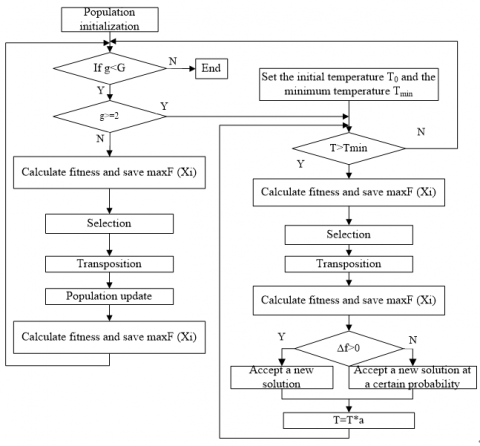Figure 4. The workflow of the SA-GA

The specific steps of solving the VRP by the SA-GA are summarized below.

Step 1. Set the initial population size NP, and generate the initial population.

According to the features of the VRP, generate a chromosome by ordinal number. First, represent the distribution set as zero, and each customer as a natural number 1-n, creating a random sequence with n numbers. Then, accumulate the customer demand from left to right. If the total demand of the first i customers exceeds the loading capacity of the vehicle, set i-1 as a breakpoint. Next, accumulate the customer demand from customer i, and set the next breakpoint. Repeat the accumulation process until reaching customer n. After that, generate a matrix of breakpoints, and add a zero at each breakpoint, the start of the sequence, and the end of the sequence. Repeat the above process of chromosome generation until the number of chromosomes reaches the population size, marking the completion of population initialization.

Step 2. Judge if the termination condition g≥G is satisfied. If yes, save the optimal solution and end the program; otherwise, go to Step 3.

Step 3. Judge if the SA operates. If g≥2, go to Step 9; otherwise, go to Step 4.

Step 4. Calculate the fitness F(Xi), and save the current optimal chromosome Xmax. If F(Xi)<F(Xi-1), go to Step (5); otherwise, go to Step (8).

Calculate fitness in the same way as traditional GA. Since the individual fitness equals the value of the objective function and the model aims to minimize the objective function, take the individual with the minimum reciprocal of fitness as the new solution to replace the optimal solution.

Step 5. Perform roulette wheel selection.

Determine the selection probability of each individual $P_{i}=F_{i} / \sum_{i=1}^{N P} F_{i}$ according to the ratio of individual fitness to global fitness. Then, generate a random number $\xi_{k} \in U(0,1) .$ If $P P_{i-1} \leq \xi_{k} \leq P P_{i}\left(P P_{i}=\sum_{j=1}^{i} P P_{j}\right)$, select individual i.

Step 6. Perform crossover and mutation to prevent illegal coding by traditional GA.

Use single parent GA to transpose one chromosome at multiple points at a preset probability Pe before crossover and mutation. Configure the transposition probability according to the number of iterations: If that number iteration time g<G/3, set the transposition probability to 0.8; if G/3≤g<2G/3, set the probability to 1-g/G; if g>2G/3, set the probability to 0.05. Then, obtain a transposition log u, which is a position in chromosome. After that, perform crossover and mutation as per the transposition probability.

Step 7. Update the population by computing individual fitness and saving the current optimal chromosome Xmax. Then, go to Step 2.

Step 8. Set the initial temperature T0 and the minimum temperature Tmin.

Step 9. If T>Tmin, repeat Steps 4-6 and then go to Step 10; otherwise, go to Step 2.

Step 10. Update the population by computing individual fitness and saving the current optimal chromosome Xmax. Then, go to Step 2. Randomly generate $\delta_{i} \in U(0,1)$ by evaluating the new solution $\Delta f=F\left(X_{i}\right)-F\left(X_{i-1}\right)>0$. If $\Delta h=\exp (\Delta f / T) \leq \delta_{i}$, accept the new solution Xi; otherwise, accept a new solution at a certain probability, and save the current solution to Min.

Step 11. Reduce the temperature Ti+1=Ti*a, and go to Step 9.

4. Example Analysis

To verify its effectiveness, the VRPSTW model optimized by SA-GA is applied to describe the collaborative distribution by three AM retailers in the downstream of the AMSC, and compared with a model not constrained by customer satisfaction. In the example, the coordinates of the three retailers are $\left[\mathrm{x}_{01}, y_{01}\right]=[4,11], \quad\left[\mathrm{x}_{02}, y_{02}\right]=[7,12]$, and $\left[\mathrm{x}_{03}, y_{03}\right]=[8,9]$, respectively; retailer 1 serves customers 1, 2, 5, 7, and 10; retailer 2 serves customers 3, 9, 12, 13, 14, and 16; retailer 3 serves customers 4, 6, 8, 11, 15, 17, and 18.

The other parameters are configured as follows: Cij = 5 yuan per vehicle per mile; $v_{0 i}=v_{i j}=60 \mathrm{miles} / \mathrm{hour} ; Q=5 ; k=1 ; \alpha=0.6,$ and $\beta=70 \%$; $\begin{array}{llll}c_{1}=10 & \text { yuan/hour; } & c_{2}=5 & \text { yuan/hour; } & t_{0}=8 & \mathrm{am} ; & d_{i j}=\end{array}$$1.2 \sqrt{\left(x_{j}-x_{i}\right)^{2}+\left(y_{j}-y_{i}\right)^{2}} .$ The location, demand, and time window of each customer are listed in Table 2.

Table 2. The locations, demands, and time windows of customers

 Customers i, j 1 2 3 4 5 6 Coodinate [xi,yi] [5,18] [0,17] [8,10] [7,15] [2,6] [10,4] Distribution quantity 1.2 1.9 0.8 1.5 1.2 0.8 [ETi,LTi] [8,12] [8,12] [8,15] [10,15] [7,14] [10,16] [ETi*,LTi*] [9,11] [10,11] [8,12] [10,11] [10,14] [12,15] Service time 0.3 0.5 0.2 0.4 0.3 0.2 Customers i, j 7 8 9 10 11 12 Coordinates [3,11] [12,6] [13,11] [5,9] [3,2] [15,16] Distribution quantity 1.6 1 1.4 0.8 1.3 1.4 [ETi,LTi] [7,12] [12,18] [12,18] [8,12] [12,18] [10,18] [ETi*,LTi*] [8,9] [12,14] [14,18] [8,9] [12,16] [13,17] Service time 0.3 0.5 0.2 0.2 0.3 0.3 Customers i, j 13 14 15 16 17 18 Coordinates [4,14] [11,15] [9,1] [12,9] [6,3] [1,10] Distribution quantity 1.5 1.3 1.2 0.9 1.1 0.8 [ETi,LTi] [8,12] [8,12] [12,18] [12,17] [12,18] [8,11] [ETi*,LTi*] [8,11] [9,11] [12,15] [12,16] [12,16] [8,9] Service time 0.4 0.3 0.4 0.3 0.4 0.2

To realize collaborative distribution, the three retailers need to set up a distribution center. The center could be a retail store or a public warehouse. The site selection must fully consider various factors, such as geographical location, traffic conditions, and inventory capacity. For simplicity, one of the three retailers was chosen as the distribution center. After comprehensive evaluation, retailer 2 was selected as the distribution center, which manages the inventory for the three retailers and serves as the starting point of all distribution tasks.

Under the above parameter setting, the optimization result and final objective function value of each route were obtained by the SA-GA. To demonstrate the algorithm performance and model reliability, a model constrained by customer satisfaction was compared with a model without that constraint.

4.1 Algorithm effectiveness

As shown in Figures 5 and 6, the optimal individual fitness decreased rapidly whether the model is constrained by customer satisfaction or not. The fitness values of the two models dropped from the initial value of 800 to 400 through about 150 iterations, and eventually converged at 436.32 and 427.67, respectively. The results demonstrate the optimization ability, optimization speed, and stability of the SA-GA.

## 5.png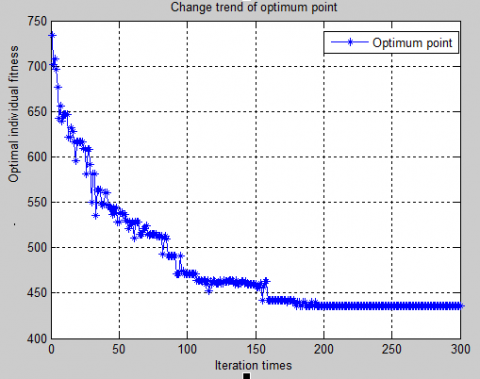Figure 5. The fitness curve of the model constrained by customer satisfaction

## 6.png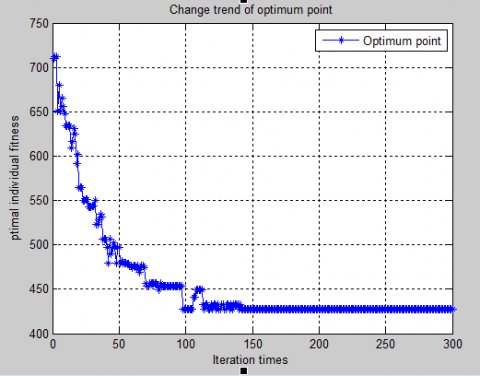Figure 6. The fitness curve of the model not constrained by customer satisfaction

4.2 Final value of objective function

As shown in Table 3, the model constrained by customer satisfaction had a total distribution cost of 663.5 yuan, a total travel distance of 129.6 miles, and an effective transport cost of 420.90 yuan. The cost incurred by the model was slightly higher than that by the model without being constrained by customer satisfaction. However, the consideration customer satisfaction reduced the penalty by 60%, and improved customer satisfaction by 49%. It is worthwhile to greatly enhance customer satisfaction at the expense of a slight growth in distribution cost. Hence, the model constrained by customer satisfaction is very effective in actual distribution.

Table 3. The comparison of optimization results

 Constrained by customer satisfaction Not constrained by customer satisfaction Total travel distance (mile) 129.60 109.80 Effective transport cost (yuan) 420.90 387.77 Penalty (yuan) 15.5 39.9 Total distribution cost (yuan) 663.50 588.90 Mean customer satisfaction 94.40% 45.40% Total service time (hour) 8.63 7.78

Table 4. The comparison between distribution plans

 Distribution route Constrained by customer satisfaction Not constrained by customer satisfaction Optimized route Carrying capacity Optimized route Carrying capacity 1 10⟶18⟶7⟶13 4.7 13⟶7⟶18 3.9 8:04 8:21 8:35 8:57 8:04 8:32 8:52 2 4⟶1⟶2 4.6 14⟶1 2⟶9⟶16 5 10:00 10:26 10:53 9:10 10:00 12:00 12:14 3 3⟶16⟶8⟶6 3.5 4⟶1⟶2 4.6 11:25 12:00 12:22 12:55 12:36 13:04 13:28 4 5⟶11⟶17⟶15 4.8 10⟶5⟶11⟶17 4.4 13:17 13:40 14:02 14:30 14:02 14:20 14:42 15:03 5 14⟶12⟶9 4.1 3⟶8⟶6⟶15 3.8 15:00 15:22 15:47 15:30 15:49 16:22 16:37

In terms of total service time, the model constrained by customer satisfaction consumed nearly 1 more hour than the model without the constraint. The main reason is the low opportunity cost of the example, which extends the waiting time and reduces the travel distance.

4.3 Optimization plan

Table 4 and Figures 7-8 compare the optimized routes, and distribution plans obtained by the models with and without the constraint of customer satisfaction. It can be seen that more than 70% of the loading capacity of vehicles were occupied in both models, and the optimized routes of the two models were highly similar. However, the two models differed greatly in the sequence of visiting customers. Despite having similar travel distances, the consideration of customer satisfaction more than doubled the customer satisfaction.

The crux of supply chain management is to put customers first, and maximize customer satisfaction. Otherwise, the demand market will not be stable or sustainable. In the short term, the model without being constrained by customer satisfaction can lower distribution cost in a certain extent. But the cost reduction is achieved against the principle and objective of supply chain management, creating a bottleneck in the long run. Thus, the model constrained by customer satisfaction is an effective way to strike a balance between cost and service.

## 7.pngFigure 7. The distribution plan constrained by customer satisfaction

## 8.png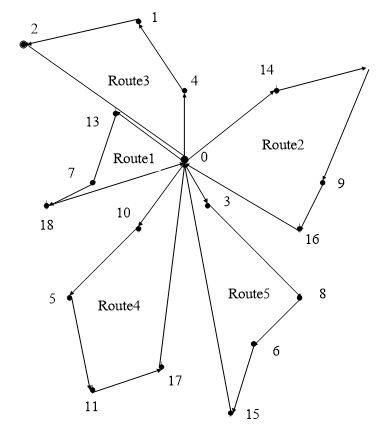Figure 8. The distribution plan not constrained by customer satisfaction

4.4 Effect of collaborative distribution

To further validate the application effect of our model, the results of collaborative distribution between the three retailers were compared with the results of separate distribution by each retailer. The results are compared in Figure 9 and Table 5.

## 9.png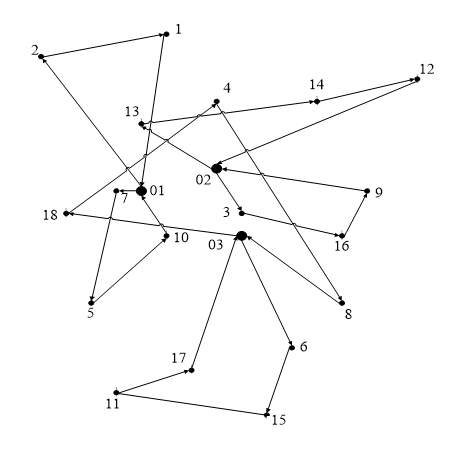Figure 9. The separate distribution plan constrained by customer satisfaction

Table 5. The comparison between optimization results

 Collaborative distribution with constraint of customer satisfaction Separate distribution plan constrained by customer satisfaction Total travel distance (mile) 129.60 144.12 Effective travel distance (mile) 84.18 101.6 Effective transport cost (yuan) 420.90 508 Penalty (yuan) 15.50 13.90 Total distribution cost (yuan) 663.50 734.50 Mean satisfaction (%) 94.40 96.40 Number of vehicles (each) 1 3 Total service time (hour) 8.63 9.04

As shown in Figure 9, the routes of the three retailers were intersected and intricate. Some routes are not economical or reasonable. By contrast, there were basically no intersected routes in collaborative distribution plan. In addition, the products occupied over 70% and even 90% of the loading capacity of vehicles in collaborative distribution with constraint of customer satisfaction, while that proportion was merely around 60% in separate distribution with constraint of customer satisfaction.

As shown in Table 5, the total distribution cost was 734.50 yuan in separate distribution with constraint of customer satisfaction, much higher than that of collaborative distribution with constraint of customer satisfaction. This is because the separate distribution has a relative long total travel distance (144.12 miles), although its penalty is relatively low. Besides, the collaborative distribution only uses 1/3 the number of vehicles adopted by separate distribution, which offsets its slightly higher penalty. The advantage of collaborative distribution is even more obvious, if considering the depreciation, repair and maintenance costs of the vehicles, and the opportunity cost incurred by idling.

In summary, the model constrained by customer satisfaction boasts a much higher customer satisfaction than the model without that constraint, despite a slightly higher total distribution cost. Moreover, the collaborative distribution of AMSC outshines separate distribution in cost, benefit, and time. Therefore, the collaborative distribution between retailers is an effective way to improve the efficiency of supply chain operations.

5. Conclusions

Targeting the collaborative distribution to multiple customers at the terminal of the AMSC, this paper sets up a collaborative distribution model, and optimizes the model with SA-GA. Then, the optimized routes of the models with and without the constraint of customer satisfaction were compared, revealing the key issues in the collaborative distribution of AMSC terminals. The main results of the research are as follows:

(1) The authors established a cost optimization model for collaborative distribution based on time window. Considering the time sensitivity of AM demand, the objective function was defined in the light of transport cost, as well as the opportunity cost and penalty incurred when the arrival time falls outside the time window required by the customer. To reflect the degree of consumer satisfaction with service time in different periods of the time window, the relationship between time window and customer satisfaction was analyzed; then, a fuzzy appointment time function was built for each customer, and treated as a constraint. By introducing that function, the objective function of total distribution cost became more realistic. In this way, the established model helps to reduce the cost of distribution enterprises, realize the goal of collaborative distribution, grasp sales opportunities in the AMSC market, and improve customer satisfaction and customer loyalty.

(2) The authors also designed a robust SA-GA. Based on the parameter features of the model, the ordinal coding method was adopted, and the single parent genetic operator was applied to perform crossover and mutation. During algorithm execution, genetic operations were implemented in the first iteration; SA operation was introduced since the second iteration. The temperature decrease was configured to control the global search and time of the GA, thereby speeding up the solving process, enhancing the convergence ability, and prevent falling into the local optimum trap.

(3) Through empirical analysis, the results of the models with and without the constraint of customer satisfaction were compared, and the distribution plan of collaborative distribution was contrasted with that of separate distribution. The comparison shows that the collaborative distribution model constrained by customer satisfaction clearly outperforms that without the constraint, and also outshines the separate distribution. Overall, the collaborative distribution model constrained by customer satisfaction boasts a much higher customer satisfaction than that without the constraint, despite its slightly higher total distribution cost, total travel distance, and effective transport cost. It is worthwhile to greatly enhance customer satisfaction at the expense of a slight growth in distribution cost. The collaborative distribution model constrained by customer satisfaction is in line with the principle of supply chain management. In addition, the collaborative distribution of AMSC is superior to separate distribution in cost, benefit, and time. The collaborative distribution is the inevitable choice for AMSC and retailers in the future.

Acknowledgment

This work was supported by Strategy and Management Base of Mineral Resources in Hebei Province, Hebei GEO University, China, and Hebei Provincial Department of Science and Technology (Grant No.: 20554703D).

References

 Aibinu A.M., Bello, S.H., Rahman, N.A., Nwohu, M.N., Akachukwu, C.M. (2016). A novel clustering based genetic algorithm for route optimization. Engineering Science and Technology, An International Journal, 19(4): 2022-2034. https://doi.org/10.1016/j.jestch.2016.08.003

 Goodarzi, A.H., Zegordi, S.H. (2016). A location-routing problem for cross-docking networks: A biogeography-based optimization algorithm. Computers & Industrial Engineering, 11(102): 132-146. https://doi.org/10.1016/j.cie.2016.10.023

 Guedria, M., Malhene, N., Deschamps, J.C. (2016). Urban freight transport: From optimized routes to robust Routes. Transportation Research Procedia, 12: 413-424. https://doi.org/10.1016/j.trpro.2016.02.076

 Wang, Y., Ma, X.L., Li, Z.B., Liu, Y., Wang, Y.H. (2017). Profit distribution in collaborative multiple centers vehicle routing problem. Journal of Cleaner Production, 15(144): 203-219. https://doi.org/10.1016/j.jclepro.2017.01.001

 Anderluh, A., Pamela, C.N., Vera, C.H., Crainic, T.G. (2019). Multi-objective optimization of a two-echelon vehicle routing problem with vehicle synchronization and ‘grey zone’ customers arising in urban logistics. European Journal of Operational Research, 289(3): 940-958. https://doi.org/10.1016/j.ejor.2019.07.049

 Tavakkoli-Moghaddam, R., Safaei, N., Gholipour, Y. (2006). A hybrid simulated annealing for capacitated vehicle routing problems with the independent route length. Applied Mathematics and Computation, 176(2): 445-454. https://doi.org/10.1016/j.amc.2005.09.040

 Pinto, T., Alves, C., Carvalho, J.V. de. (2020). Variable neighborhood search algorithms for the vehicle routing problem with two-dimensional loading constraints and mixed linehauls and backhauls. International Transactions in Operational Research, 27(1): 549-572. https://doi.org/10.1111/itor.12509

 Archetti, C., Speranza, M.G., Boccia, M., Sforza, A., Sterl, C. (2020). A branch-and-cut algorithm for the inventory routing problem with pickups and deliveries. European Journal of Operational Research, 282(3): 886-895. https://doi.org/10.1016/j.ejor.2019.09.056

 Lmariouh, J., Hachemi, N., El Jamali M.A., Bouami, D., Martin, R.L. (2019). An integrated production and distribution problem with direct shipment: A case from Moroccan bottled-water market. International Journal of Operational Research, 34(1): 144-160. https://doi.org/10.1504/IJOR.2019.096942

 Li, J.H., Guo, H., Zhou, Q.H., Yang, B.X. (2019). Vehicle routing and scheduling optimization of ship steel distribution center under green shipbuilding mode. Sustainability, 11(15): 4248. https://doi.org/10.3390/su11154248

 Bruni, M.E., Berald, P., Khodaparasti, S. (2018). A fast heuristic for routing in post-disaster humanitarian relief logistics. Transportation Research Procedia, 30: 304-313. https://doi.org/10.1016/j.trpro.2018.09.033

 McLeod, F.N., Cherrett, T.J., Bektas, T., Allen, J., Martinez-Sykora, A., Lamas-Fernandez, C., Bates, O., Cheliotis, K., Friday, A., Piecyk, M., Wise, S. (2020). Quantifying environmental and financial benefits of using porters and cycle couriers for last-mile parcel delivery. Transportation Research Part D: Transport and Environment, 82: 102311. https://doi.org/10.1016/j.trd.2020.102311

 Zulvia, F.E., Kuo, R.J., Nugroho, D.Y. (2020). A many-objective gradient evolution algorithm for solving a green vehicle routing problem with time windows and time dependency for perishable products. Journal of Cleaner Production, 242: 118428. https://doi.org/10.1016/j.jclepro.2019.118428

 Zhao, Z.X., Li, X.M., Zhou, X.C., (2020). Distribution route optimization for electric vehicles in urban cold chain logistics for fresh products under time-varying traffic conditions. Mathematical Problems in Engineering. https://doi.org/10.1155/2020/9864935

 Ricardo, P.R., Arturo, H.A. (2019). A hybrid estimation of distribution algorithm for the vehicle routing problem with time windows. Computers & Industrial Engineering, 130: 75-96. https://doi.org/10.1016/j.cie.2019.02.017

 Bezerra, S.N., de Souza, S.R., Souza, M.J.F. (2018). A GVNS algorithm for solving the multi-depot vehicle routing problem. Electronic Notes in Discrete Mathematics, 66: 167-174. https://doi.org/10.1016/j.endm.2018.03.022.

 Yahyaoui, H., Krichen, S., Dekdouk, A. (2018). A Decision Model Based on a GRASP Genetic Algorithm for Solving the Vehicle Routing Problem. International Journal of Applied Metaheuristic Computing (IJAMC), 9(2): 72-90. https://doi.org/10.4018/IJAMC.2018040104

 Christos, O., Demetrio, L., Wout, D., Daniele, V. (2020). Distribution with quality of service considerations: the capacitated routing problem with profits and service level requirements. Omega, 93: 102034. https://doi.org/10.1016/j.omega.2019.02.003

 Hanafi, R., Rusman, M., Mardin, F., Parenreng, S.M., Azzazli, A. (2020). Distribution route optimization of a capacitated vehicle routing problem by sweep algorithm. IOP Conference Series: Materials Science and Engineering, 875(1): 012066. https://doi.org/10.1088/1757-899X/875/1/012066

 Danchuk, V., Bakulich, O., Svatko, V. (2017). An improvement in ant algorithm method for optimizing a transport route with regard to traffic flow. Procedia Engineering, 187: 425-434. https://doi.org/10.1016/j.proeng.2017.04.396

 Zhang, Z.Z., Wang, L.H. (2017). Application of improved artificial bee colony algorithm in urban vegetable distribution route optimization. Journal of Applied Mathematics and Physic, 5(11): 2291-2301. https://doi.org/10.4236/jamp.2017.511186

 Schermer, D., Moeini M., Wendt O. (2019). A hybrid VNS/Tabu search algorithm for solving the vehicle routing problem with drones and en route operations. Computers and Operations Research, 109: 134-158. https://doi.org/10.1016/j.cor.2019.04.021.

 Goel, R., Maini, R. (2020). Evolutionary ant colony algorithm using firefly based transition for solving vehicle routing problems: EAFA for VRPs. International Journal of Swarm Intelligence Research (IJSIR), 10(3): 46-60. https://doi.org/10.4018/IJSIR.2019070103

 Sicilia, J.A., Quemada, C., Royo, B., Escuín, D. (2016). An optimization algorithm for solving the rich vehicle routing problem based on Variable Neighborhood Search and Tabu Search metaheuristics. Journal of Computational and Applied Mathematics, 1(291): 468-477. https://doi.org/10.1016/j.cam.2015.03.050

 Wang, X.P., Wang, M., Ruan, J.H., Zhan, H.X. (2016). The multi-objective optimization for perishable food distribution route considering temporal-spatial distance. Procedia Computer Science, (96): 1211-1220. https://doi.org/10.1016/j.procs.2016.08.165

 Singh, V., Ganapathy, L., Ashok, K.P. (2019). An improved genetic algorithm for solving multi depot vehicle routing problems. International Journal of Information Systems and Supply Chain Management (IJISSCM), 12(4): 1-26. https://doi.org/10.4018/IJISSCM.2019100101